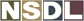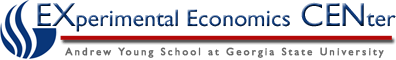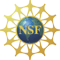Students / SubjectsHandbook >> Supply >>

# Using an Equation

Although tables and graphs are very useful in representing the supply for a product, equations are sometimes used as well. Although many students try to avoid using equations, this is actually the easiest (and shortest) way to represent the supply for a product. For example, what if I wanted to know how many cases of wine producers would be willing to sell if the price of a bottle of wine were \$21? Getting the precise number from the table may be difficult. It would require extrapolating from the points that are there. On the other hand, if I gave you an equation it would be very easy.

Suppose I told you the supply for red wine was represented by the following equation:

Q = 5,000 + 2,000*P

First, let's look at the prices we've already used. If the price of a bottle is \$10, just insert that into this equation:

Q = 5,000 + 2,000(10)
Q = 5,000 + 20,000
Q = 25,000

This is the same as we see in our first table (and in the first figure).

If the price of a bottle of wine were \$20, we would have:

Q = 5,000 + 2,000(20)
Q = 5,000 + 40,000
Q = 45,000

If something other than the price of a bottle of wine were to change, this would mean the equation itself would change. For example, the supply for wine after the new machines were released is:

Q = 5,000 + 2,000P + 30,000
Q = 35,000 + 2,000P

So if the price of a bottle of wine were \$10, we would have:

Q = 35,000 + 2,000(10)
Q = 35,000 + 20,000
Q = 55,000

You can see for yourself you get the other entries in our table (or graph) if the price of a bottle of wine were \$20 or \$30. As before, this is the same number we had before.

We can now easily use this equation to determine the quantity supplied of wine if the price of a bottle were \$21:

Q = 5,000 + 2,000(21)
Q = 5,000 + 42,000
Q = 47,000

Back to Supply